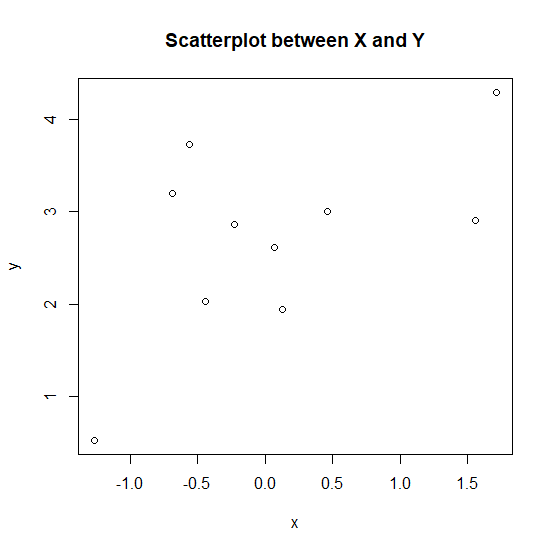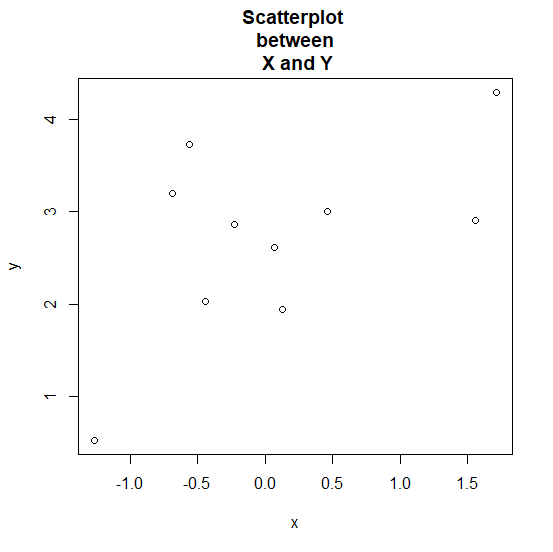# How to write the plot title in multiple lines using plot function in R?

Mostly, the main title of a plot is short but we might have a long line to write for the main title of the plot. For example, a short version might be “Scatterplot” and a longer version might be “Scatterplot between X and Y”. Therefore, in plot function of R we can use line breaks for the main title as "Scatterplot
between
X and Y".

## Example

set.seed(123)
x <-rnorm(10)
y <-rnorm(10,2.5)

Creating a simple scatterplot between x and y with main title −

plot(x,y,main="Scatterplot between X and Y")

## OutputCreating a simple scatterplot between x and y with main title in multiple lines −

## Output

plot(x,y,main="Scatterplot  between  X and Y")

## Output